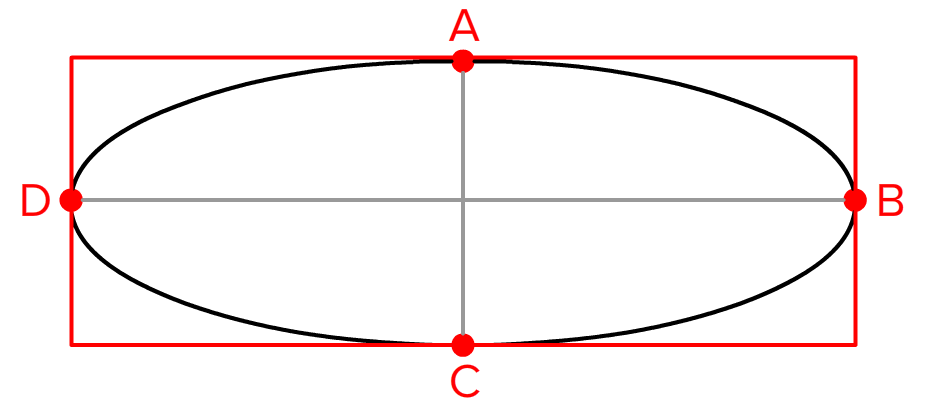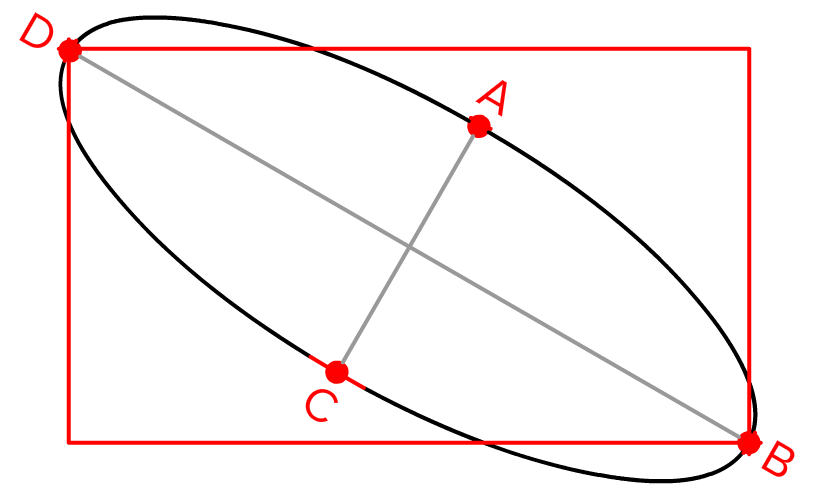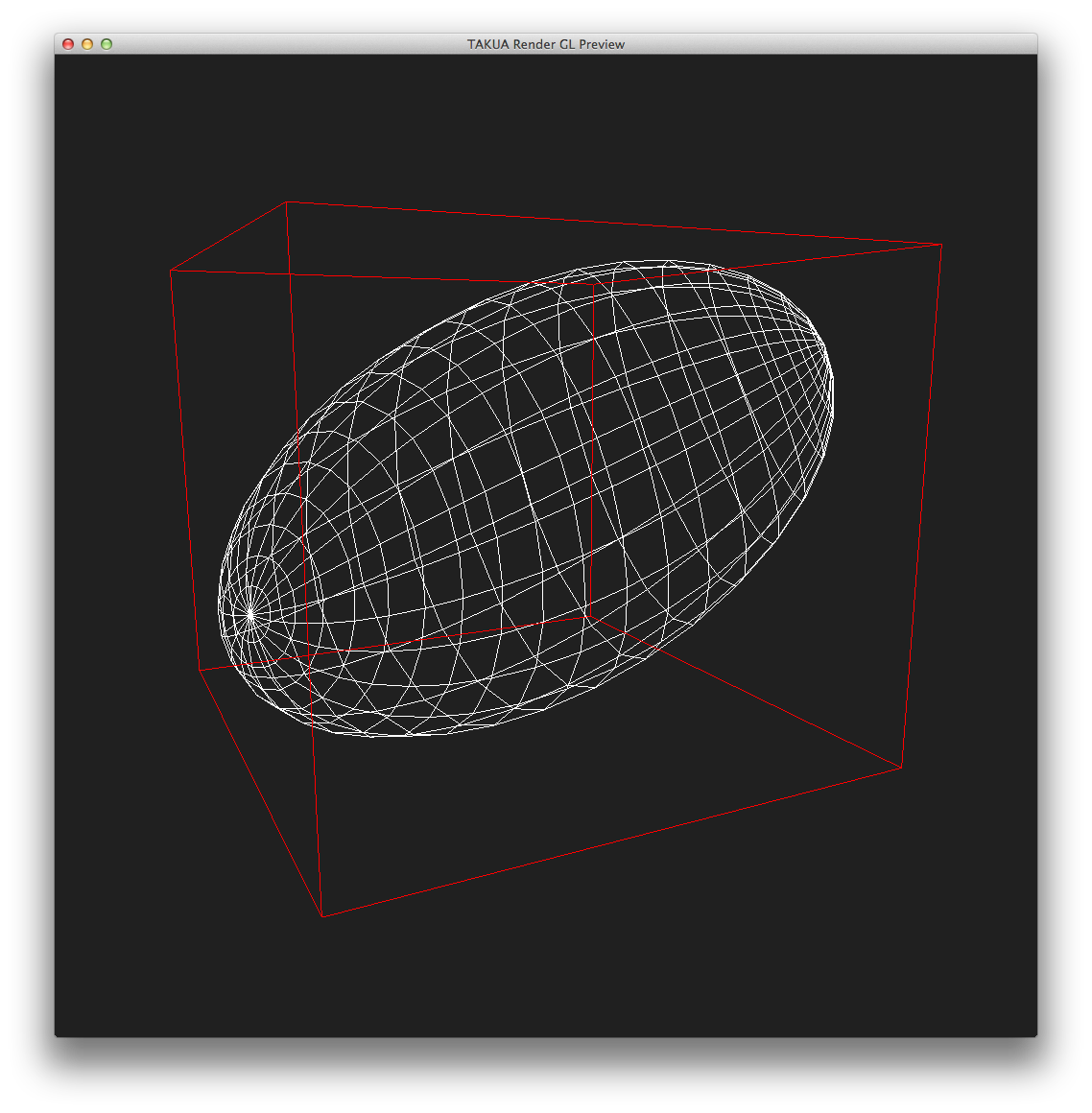## Bounding Boxes for Ellipsoids

Warning: this post is going to be pretty math-heavy.

Let’s talk about spheres, or more generally, ellipsoids. Specifically, let’s talk about calculating axis aligned bounding boxes for arbitrarily transformed ellipsoids, which is a bit of an interesting problem I recently stumbled upon while working on Takua Rev 3. I’m making this post because finding a solution took a lot of searching and I didn’t find any single collected source of information on this problem, so I figured I’d post it for both my own reference and for anyone else who may find this useful.

So what’s so hard about calculating tight axis aligned bounding boxes for arbitrary ellipsoids?

Well, consider a basic, boring sphere. The easiest way to calculate a tight axis aligned bounding box (or AABB) for a mesh is to simply min/max all of the vertices in the mesh to get two points representing the min and max points of the AABB. Similarly, getting a tight AABB for a box is easy: just use the eight vertices of the box for the min/max process. A naive approach to getting a tight AABB for a sphere seems simple then: along the three axes of the sphere, have one point on each end of the axis on the surface of the sphere, and then min/max. Figure 1. shows a 2D example of this naive approach, to extend the example to 3D, simply add two more points for the Z axis (I drew the illustrations quickly in Photoshop, so apologies for only showing 2D examples):This naive approach, however, quickly fails if we rotate the sphere such that its axes are no longer lined up nicely with the world axes. In Figure 2, our sphere is rotated, resulting in a way too small AABB if we min/max points on the sphere axes:If we scale the sphere such that it becomes an ellipsoid, the same problem persists, as the sphere is just a subtype of ellipsoid. In Figures 3 and 4, the same problem found in Figures 1/2 is illustrated with an ellipsoid:One possible solution is to continue using the naive min/max axes approach, but simply expand the resultant AABB by some percentage such that it encompasses the whole sphere. However, we have no way of knowing what percentage will give an exact bound, so the only feasible way to use this fix is by making the AABB always larger than a tight fit would require. As a result, this solution is almost as undesirable as the naive solution, since the whole point of this exercise is to create as tight of an AABB as possible for as efficient intersection culling as possible!

Instead of min/maxing the axes, we need to use some more advanced math to get a tight AABB for ellipsoids.

We begin by noting our transformation matrix, which we’ll call M. We’ll also need the transpose of M, which we’ll call MT. Next, we define a sphere S using a 4x4 matrix:

[ r 0 0 0 ]
[ 0 r 0 0 ]
[ 0 0 r 0 ]
[ 0 0 0 -1]


where r is the radius of the sphere. So for a unit diameter sphere, r = .5. Once we have built S, we’ll take its inverse, which we’ll call SI.

We now calculate a new 4x4 matrix R = M*SI*MT. R should be symmetric when we’re done, such that R = transpose(R). We’ll assign R’s indices the following names:

R = [ r11 r12 r13 r14 ]
[ r12 r22 r23 r24 ]
[ r13 r23 r23 r24 ]
[ r14 r24 r24 r24 ]


Using R, we can now get our bounds:

zmax = (r23 + sqrt(pow(r23,2) - (r33*r22)) ) / r33;
zmin = (r23 - sqrt(pow(r23,2) - (r33*r22)) ) / r33;
ymax = (r13 + sqrt(pow(r13,2) - (r33*r11)) ) / r33;
ymin = (r13 - sqrt(pow(r13,2) - (r33*r11)) ) / r33;
xmax = (r03 + sqrt(pow(r03,2) - (r33*r00)) ) / r33;
xmin = (r03 - sqrt(pow(r03,2) - (r33*r00)) ) / r33;


…and we’re done!

Just to prove that it works, a screenshot of a transformed ellipse inside of a tight AABB in 3D from Takua Rev 3’s GL Debug view:I’ve totally glossed over the mathematical rationale behind this method in this post and focused just on how to quickly get a working implementation, but if you want to read more about the actual math behind how it works, these are the two sources I pulled this from:

Stack Overflow post by user fd

Article by Inigo Quilez

In other news, Takua Rev 3’s new scene system is now complete and I am working on a brand new, better, faster, stackless KD-tree implementation. More on that later!